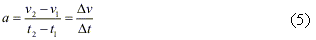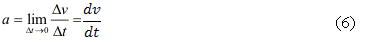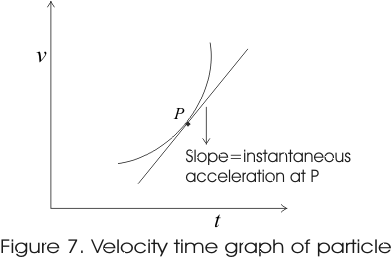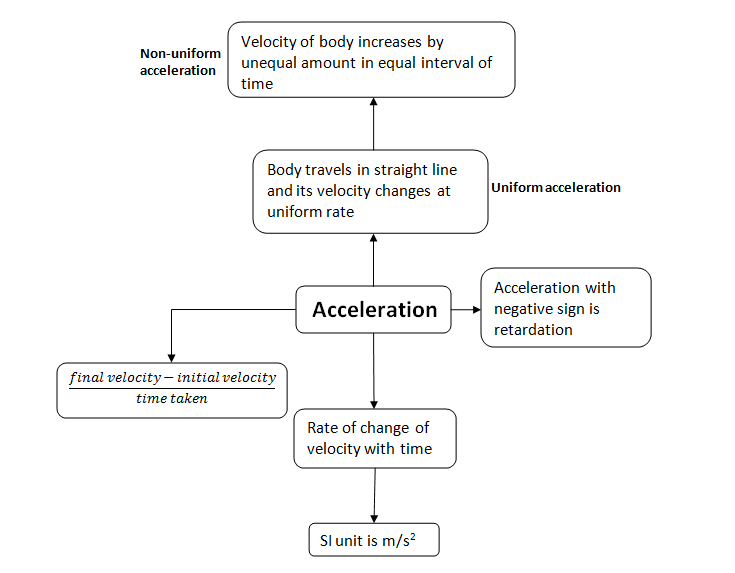# Acceleration, definition, formula and deceleration

## 5. Acceleration

• Acceleration is the rate of change of velocity with time.
• For describing average acceleration we first consider the motion of an object along X-axis.
• Suppose at time t1 object is at point P moving with velocity v1 and at time t2 it is at point Q and has velocity v2. Now average acceleration of object in moving from P to Q iswhich is the change in velocity of object with the passage of time.
• Instantaneous acceleration can be defined in the same way as instantaneous velocity• The instantaneous acceleration at any instant is the slope of v-t graph at that instant.• In figure 7 instantaneous acceleration at point P is equal to the slope of tangent at this point P.
• Since velocity of a moving object has both magnitude and direction likewise acceleration depending on velocity has both magnitude and direction and hence acceleration is a vector quantity.
• Acceleration can also be positive, negative and zero.
• SI unit of acceleration is ms-2

### Acceleration Concept MapQuestion:
A car moves in a straight road. The velocity at point A is 50 km/hr, It presses the accelerator paddle and reach point B in 10 sec. The velocity at point B is 100Km/hr
Find the average acceleration of the car from point A to point B?
Solution
Initial Velocity =50km/hr= 13.88 m/s
Final Velocity = 100Km/hr= 27.77 m/s
Average Acceleration= Change in velocity/time taken= (27.77-18.88)/10=1.389 m/s/2
Question:
True and False Statement
a) Acceleration is a scalar quantity?
b) if the velocity of the object is zero,then acceleration will always be zero?
c) Instantaneous speed is always equal to magnitude of Instantaneous velocity
Solution
a) False
b) False
c) True